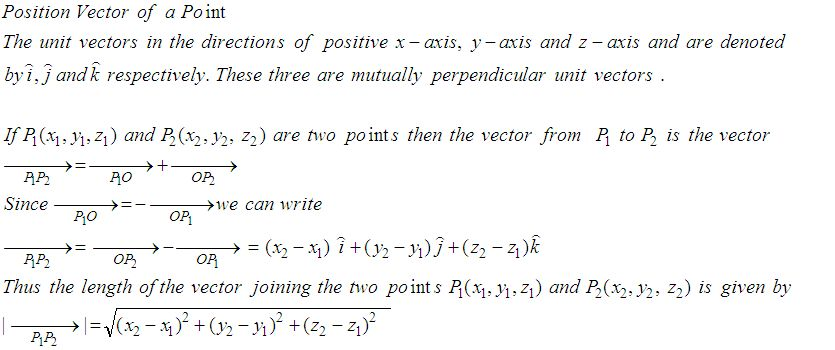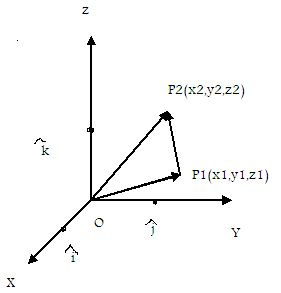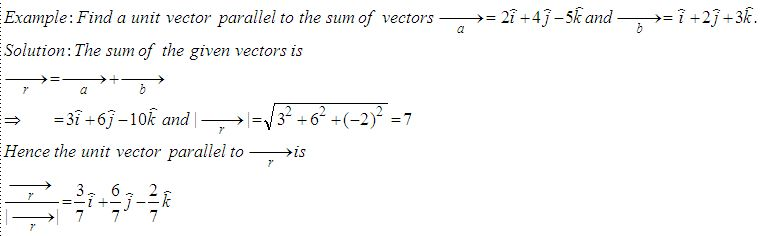Email us to get an instant 20% discount on highly effective K-12 Math & English kwizNET Programs!

Online Quiz (WorksheetABCD)

Questions Per Quiz = 2 4 6 8 10

High School Mathematics - 25.9 Position Vector of a PointDirections: Answer the following.
 Q 1: If P = 2,4,7 and P2 = (-4, -1, 5) then find vector P1P2.-6i+5j-6i-5j-2k6i+5j+3k Q 2: Find a unit vector parallel to the sum of vectors a = 2i+4j-5k, b = i+2j+3k2-i83/7i + 6/7j - 2/7 k8i+4j-3k Q 3: Find the unit vector in the direction of P(1,2,3) towards Q(4,5,6).6i+5j+2k8j-2k(i+j+k)/Ö3 Q 4: Find the unit vector in the direction of of P(1,2) and Q(4,5).3i-2j(i+j)/Ö22i+5j Q 5: Find the vector with initial point P(6,-2) and terminal point Q(4,-8).8i+2j-2i+10j6i-9j Q 6: Find the condition that the vectors a = ki + 3j and b = 4i+kj, (k not equal to 0) are parallel.k2 = 12k = 6k = 0 Question 7: This question is available to subscribers only! Question 8: This question is available to subscribers only!